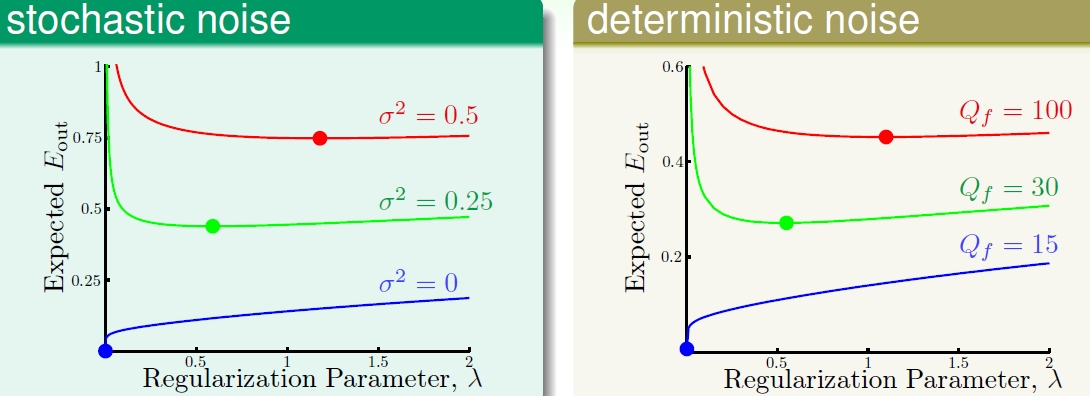台大-林轩田老师-机器学习基石学习笔记14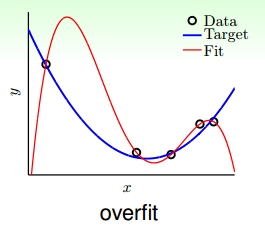step back不仅用于篮球中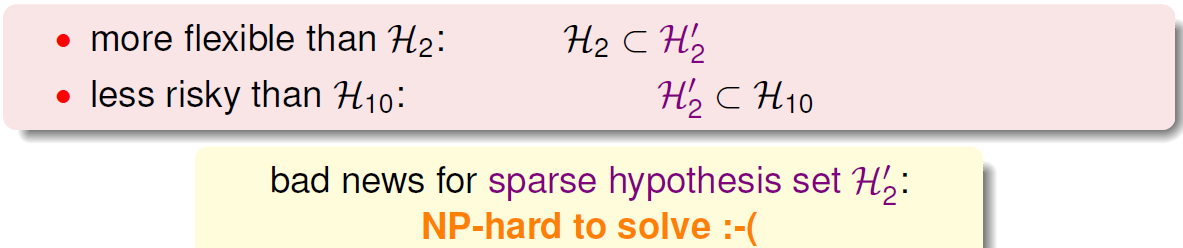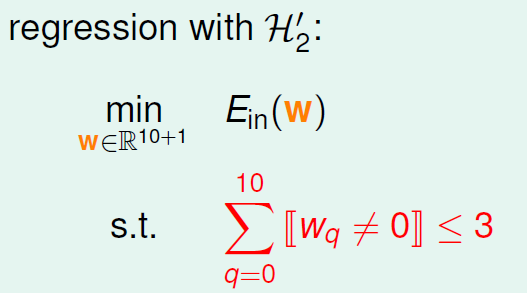${H}_{10}$$H_{10}$=${w}_{0}$$w_{0}$+${w}_{1}{x}^{1}$$w_{1}x^{1}$x+${w}_{2}{x}^{2}$$w_{2}x^{2}$+${w}_{3}{x}^{3}$$w_{3}x^{3}$+⋯+${w}_{10}{x}^{10}$$w_{10}x^{10}$

${H}_{2}$$H_{2}$可表示为：

${H}_{2}$$H_{2}$=${w}_{0}$$w_{0}$+${w}_{1}{x}^{1}$$w_{1}x^{1}$x+${w}_{2}{x}^{2}$$w_{2}x^{2}$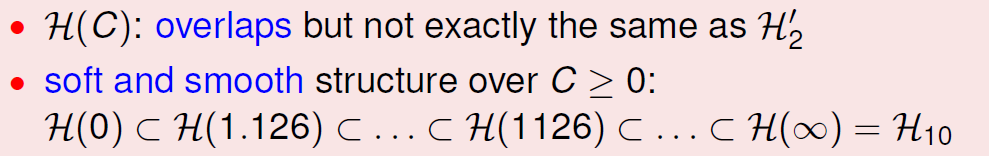我们需要有个上限

$\sum ‖{w}^{2}‖$\sum \left \| w^{2} \right \|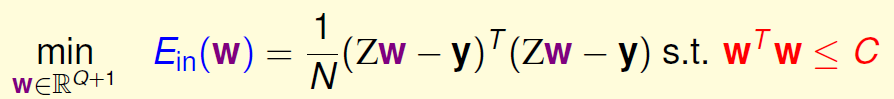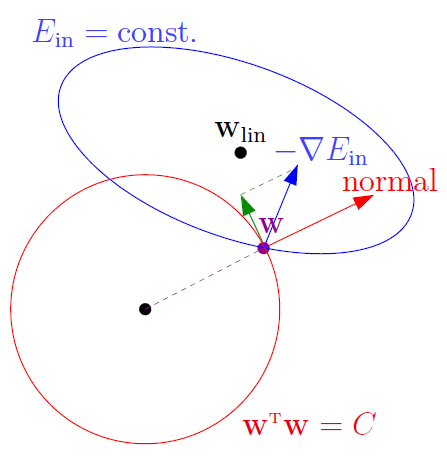$▽{E}_{in}\left({W}_{REG}\right)+\frac{2\lambda }{N}\cdot {W}_{REG}=0$$\triangledown E_{in}(W_{REG})+\frac{2\lambda }{N} \cdot W_{REG}=0$
λ称为Lagrange multiplier，是用来解有条件的最佳化问题常用的数学工具
$\frac{2}{N}$$\frac{2}{N}$是方便后面公式推导。那么我们的目标就变成了求解满足上面公式的${w}_{REG}$$w_{REG}$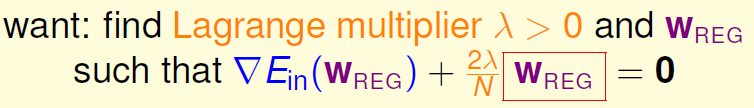${W}_{REG}=\left({Z}^{T}\cdot Z+\lambda I{\right)}^{-1}\cdot {Z}^{T}\cdot y$$W_{REG}=(Z^{T}\cdot Z +\lambda I)^{-1}\cdot Z^{T}\cdot y$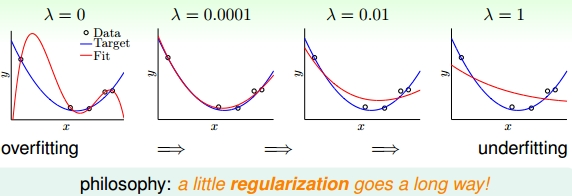好像和VC理论有一定的联系

Augmented Error表达式如下：

${E}_{aug}\left(W\right)={E}_{in}\left(W\right)+\frac{\lambda }{N}\cdot {W}^{T}\cdot W$$E_{aug}(W)=E_{in}(W)+\frac{\lambda}{N}\cdot W^{T}\cdot W$

${E}_{out}\le {E}_{in}+\mathrm{\Omega }\left(H\right)$$E_{out}\leq E_{in}+\Omega(H)$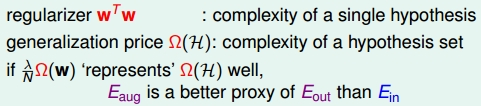最后归纳总结通用的正则化

1. target-dependent
2. plausible
3. friendly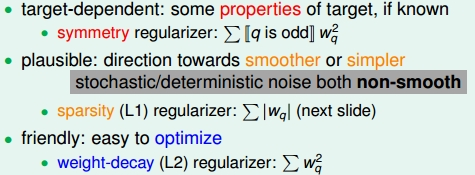这个误差的测量一样也有三种一模一样的方式：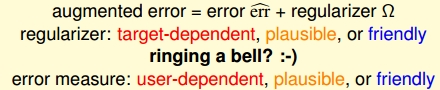在详细说一下$\lambda$$\lambda$的取值：